The QLIM Procedure

Naming

Subsections:

Naming of Parameters

When there is only one equation in the estimation, parameters are named in the same way as in other SAS procedures such as REG, PROBIT, and so on. The constant in the regression equation is called Intercept. The coefficients on independent variables are named by the independent variables. The standard deviation of the errors is called _Sigma. If there are Box-Cox transformations, the coefficients are named _Lambdai, where i increments from 1, or as specified by the user. The limits for the discrete dependent variable are named _Limiti. If the LIMIT=varying option is specified, then _Limiti starts from 1. If the LIMIT=varying option is not specified, then _Limit1 is set to 0 and the limit parameters start from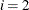. If the HETERO statement is included, the coefficients of the independent variables in the hetero equation are called _H.x, where x is the name of the independent variable. If the parameter name includes interaction terms, it needs to be enclosed in quotation marks followed by
. The following example restricts the parameter that includes the interaction term to be greater than zero:

   proc qlim data=a;
model y = x1|x2;
endogenous y ~ discrete;
restrict "x1*x2"N>0;
run;


When there are multiple equations in the estimation, the parameters in the main equation are named in the format of y.x, where y is the name of the dependent variable and x is the name of the independent variable. The standard deviation of the errors is called _Sigma.y. The correlation of the errors is called _Rho for bivariate model. For the model with three variables it is _Rho.y1.y2, _Rho.y1.y3, _Rho.y2.y3. The construction of correlation names for multivariate models is analogous. Box-Cox parameters are called _Lambdai.y and limit variables are called _Limiti.y. Parameters in the HETERO statement are named as _H.y.x. In the OUTEST= data set, all variables are changed from ’.’ to ’_’.

Naming of Output Variables

The following table shows the option in the OUTPUT statement, with the corresponding variable names and their explanation.

Option

Name

Explanation

PREDICTED

P_y

Predicted value of y

RESIDUAL

RESID_y

Residual of y, (y-PredictedY)

XBETA

XBETA_y

Structure part (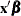) of y equation

ERRSTD

ERRSTD_y

Standard deviation of error term

PROB

PROB_y

Probability that y is taking the observed value in this observation (discrete y only)

PROBALL

PROBi_y

Probability that y is taking the ith value (discrete y only)

MILLS

MILLS_y

Inverse Mills ratio for y

EXPECTED

EXPCT_y

Unconditional expected value of y

CONDITIONAL

CEXPCT_y

Conditional expected value of y, condition on the truncation.

MARGINAL

MEFF_x

Marginal effect of x on y (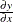) with single equation

MEFF_y_x

Marginal effect of x on y () with multiple equations

MEFF_Pi_x

Marginal effect of x on y (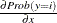) with single equation and discrete y

MEFF_Pi_y_x

Marginal effect of x on y () with multiple equations and discrete y

TE1

TE1

Technical efficiency estimate for each producer proposed by Battese and Coelli (1988)

TE2

TE2

Technical efficiency estimate for each producer proposed by Jondrow et al. (1982)

If you prefer to name the output variables differently, you can use the RENAME option in the data set. For example, the following statements rename the residual of y as Resid:

   proc qlim data=one;
model y = x1-x10 / censored;
output out=outds(rename=(resid_y=resid)) residual;
run;